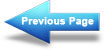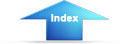Custom SearchDesign: Solar Heating of Buildings and Domestic Hot WaterMIL-HDBK-1003/13A
For 7% discount, from Table 3-5c
Present worth
=
12.057 x value of fuel saved/yr - 0% inflation
=
19.931 x value of fuel saved/yr - 5% inflation
=
28.146 x value of fuel saved/yr - 8% inflation
Use these values for Worksheet E-1, column (6).
The inflation rate for fuel
oil, natural gas and LPG is currently 8%.
LIFETIME O&M COSTS - (WORKSHEET E-1)
The present worth of these costs are calculated the same as the fuel savings,
except zero inflation is assumed.
LIFETIME O&M COSTS = Cost determined
x (PWF)
from Section 3.7.2
For 10% discount, 0% inflation, 25 years, the present worth is 9.52 times the
cost (from Table 3-5a).
For 7% discount, 0% inflation, 25 years, the present worth is 12.057 times
the cost (from Table 3-5c).  Use these factors for Worksheet E-1, column (8).
The net fuel savings (Lifetime fuel savings minus Lifetime O&M costs) are
computed on Worksheet E-1, column (9) and transferred to Worksheet A, column
(y) to compute SIR.  Complete for each collector area under study.
3.7.3.2 Present worth analysis without the discount factor - Worksheet E-1.
This method was recommended by NAVFACINST 11010.14M, 14 December 1978, and
may still be applicable to some projects.  This method generally calculates
the cost effectiveness in the "most favorable" way.  Hence, if such a
calculation is desired this method would be the choice.  DoD policy will
specify the method to be used, therefore this method should only be used in
the appropriate circumstances.  Generally O&M costs are set equal to zero in
this method; therefore, column (8) of Worksheet E-1 should equal zero.
However, the user may assume an O&M cost and it is recommended to do so
especially in high maintenance systems.  Flat plate systems are assumed to
have 0% O&M for this method.
The calculation method is the same as Section 3.7.3 (with a discount factor)
except that the present worth factors are from Table 3-5b and as noted above
the O&M costs are usually equal to zero.  For 25 years lifetime, and 8%
inflation rate the present worth factor for Worksheet E-1, column (7) is
78.954.  The present worth of the net fuel savings, Worksheet E-1, column
(9), are transferred to Worksheet A, column (y).  Complete for each collector
area under study.
3.8 Collector temperatures - Worksheet E-1.  Fluid temperature rise through
collector is of interest and may be calculated from:
(f)(QL)(No)
To - Ti
G Cp [theta] Ac
111Integrated Publishing, Inc. - A (SDVOSB) Service Disabled Veteran Owned Small Business